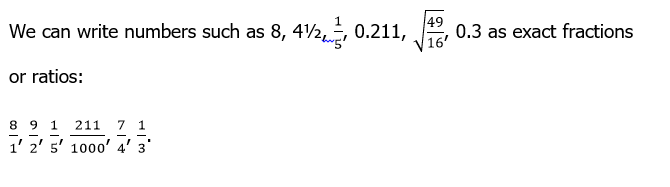### Rational and non rational numbers 2

Mathematics J.S.S 3 First Term

Theme: Number and Numeration

Sub Theme: Whole Number

WEEK 9

Rational and non-rational numbers 2

Performance Objectives

Students should be able to;

1. Approximating numbers to significant places
2. Approximate numbers to any degree of accuracy
3. Solve quantitative reasoning problems related to the approximation of numbers

Square Root of Numbers

ContentSuch numbers are called rational numbers.

Numbers which cannot be written as exact fractions are called non-fractional numbers, or irrational numbers. √7 is an example of a non-rational number. √7 = 2.645 751 …., the decimals extending without end and without recurring.

π is another example of a non-rational number. Π = 3.141 592 …., again extending forever without repetition. The fraction View More